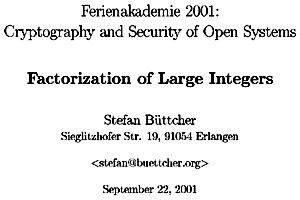Abstract

Modern public-key cryptosystems are based on modular arithmetic. The security of many of them relies on the intractability of the Factorization Problem, i.e. the difficulty to compute the prime factors of a given integer. This gives some motivation to study the possibilities of computing the prime factor decomposition of an integer.

In this essay, the early attempts (Trial Division, Fermat's method) as well as Pollard's p-1 and Rho algorithms are covered. Its main focus, however, lies on Pomerance's Quadratic Sieve algorithm, which lead to the Number Field Sieve, today's most powerful factorization algorithm.

Java Sources of Trial Division, Fermat, p-1, Rho, QS and CFRAC have been added as well.

Available resources